# NCERT Solutions, Determinants, Part -5, Class 12, Maths, Notes - JEE

## JEE: NCERT Solutions, Determinants, Part -5, Class 12, Maths, Notes - JEE

The document NCERT Solutions, Determinants, Part -5, Class 12, Maths, Notes - JEE is a part of JEE category.
All you need of JEE at this link: JEE

Determinants

Exercise 4.5

Question 1: Find adjoint of each of the matrices.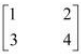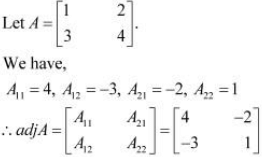Question 2: Find adjoint of each of the matrices.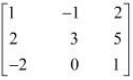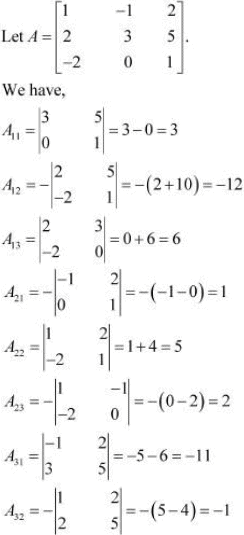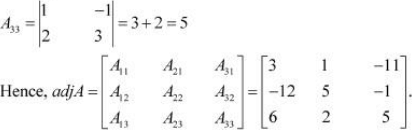Question 3: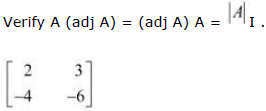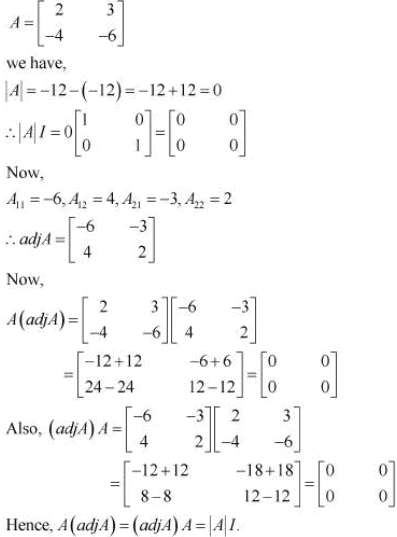Question 4: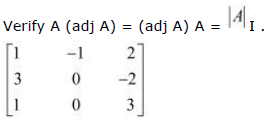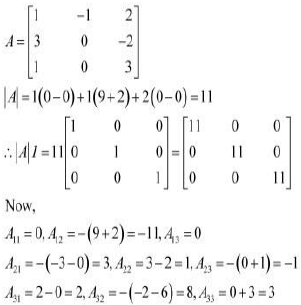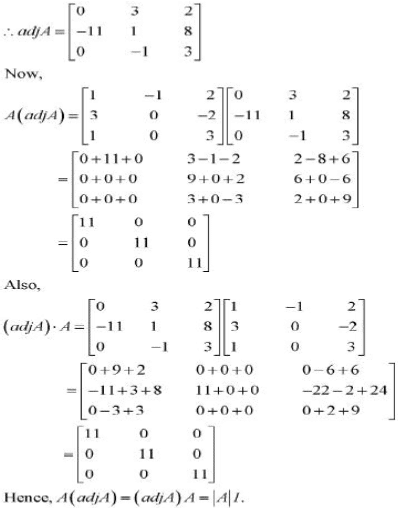Question 5: Find the inverse of each of the matrices (if it exists).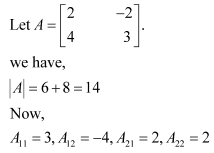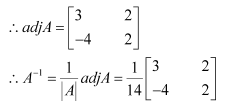Question 6: Find the inverse of each of the matrices (if it exists).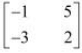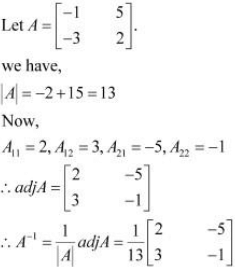Question 7: Find the inverse of each of the matrices (if it exists).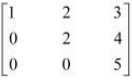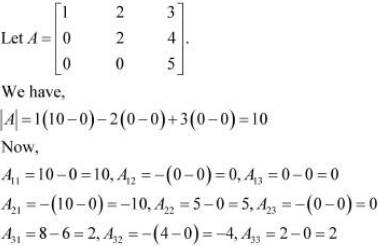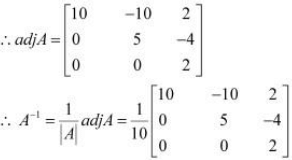Question 8: Find the inverse of each of the matrices (if it exists).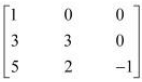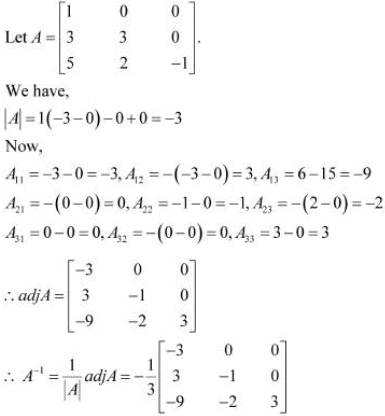Question 9: Find the inverse of each of the matrices (if it exists).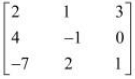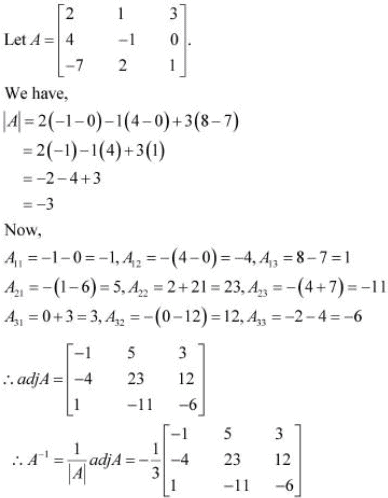Question 10: Find the inverse of each of the matrices (if it exists).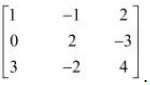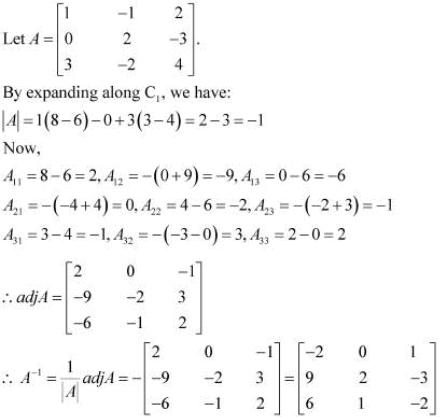Question 11: Find the inverse of each of the matrices (if it exists).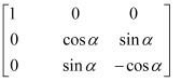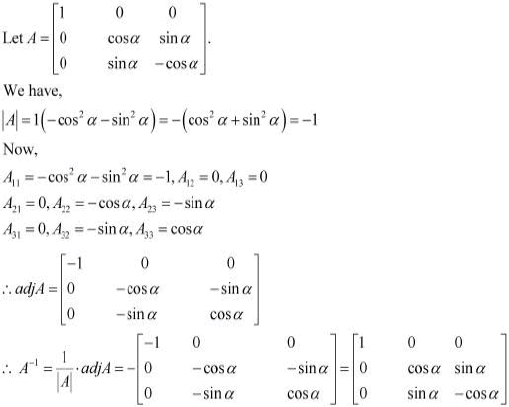Question 12:
Let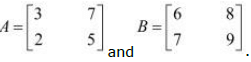. Verify that (AB)-1 = B-1A-1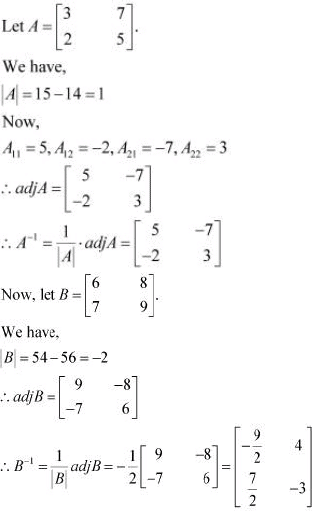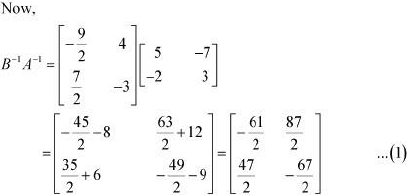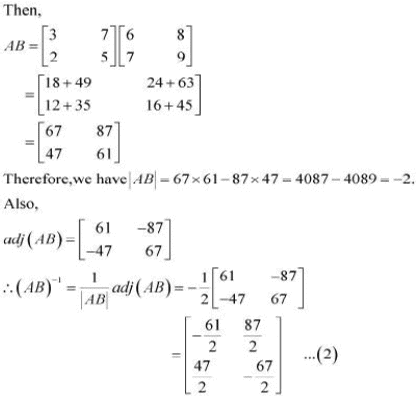From (1) and (2), we have:
(AB)−1 = B−1A−1
Hence, the given result is proved.

Question 13:
If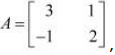show that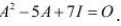Hence find A-1.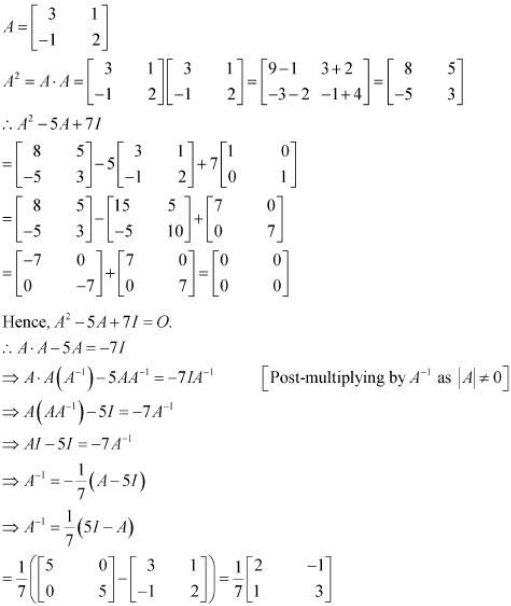Question 14:
For the matrix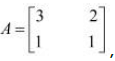, find the numbers a and b such that A2 + aA + bI = O.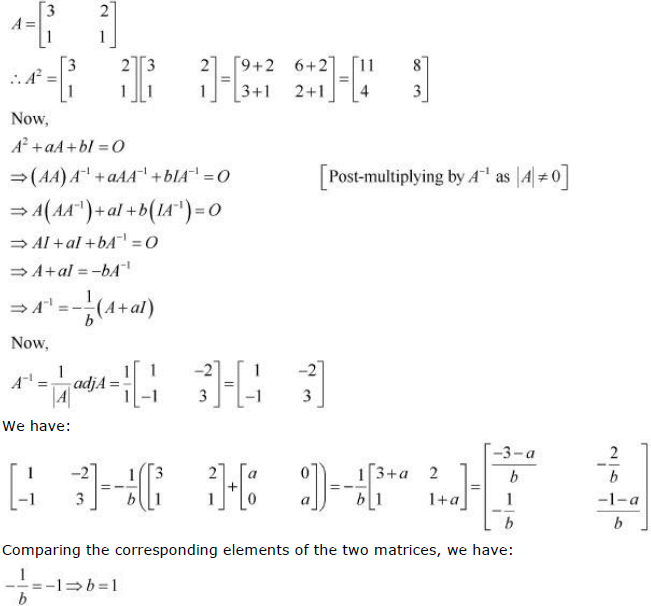Hence, −4 and 1 are the required values of a and b respectively.

Question 15:
For the matrix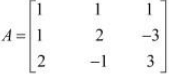show that A3 − 6A2 + 5A + 11 I = O. Hence, find
A−1.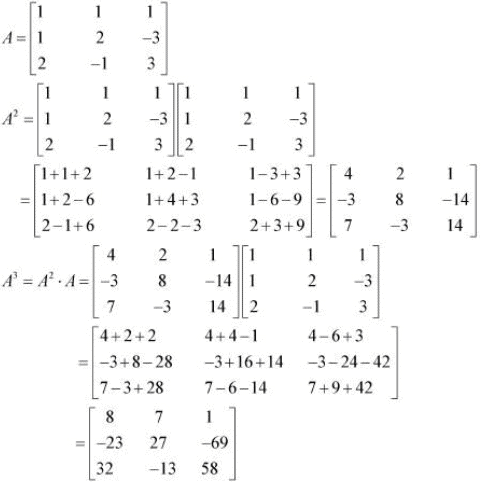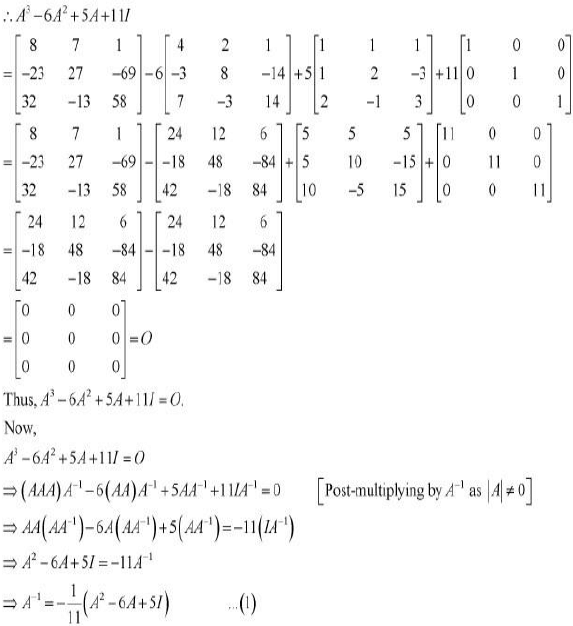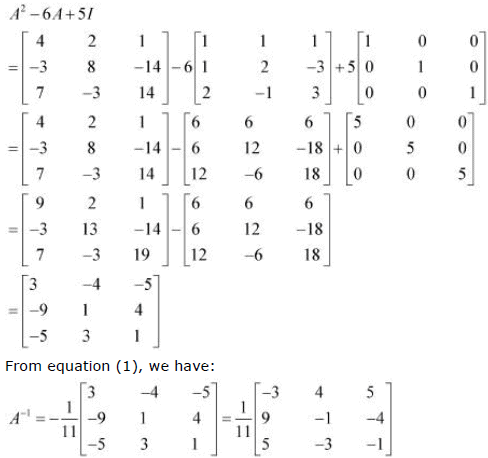Question 16:
If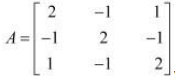verify that A3 − 6A2 + 9A − 4I = O and hence find A−1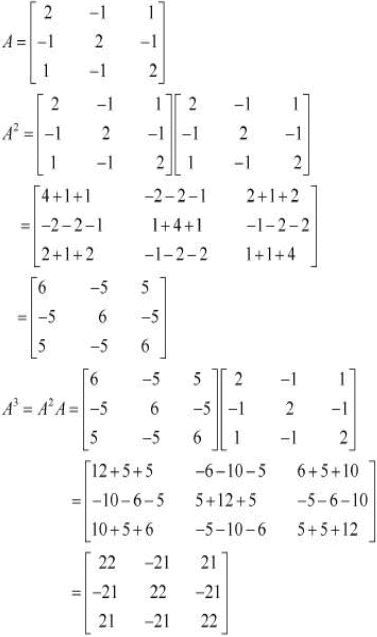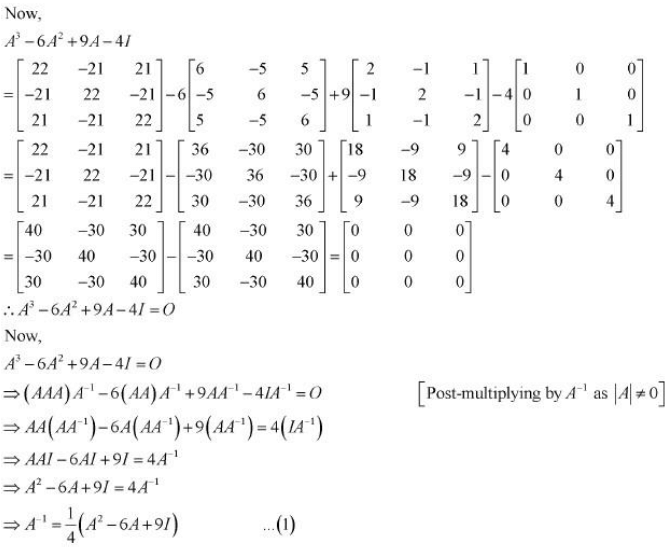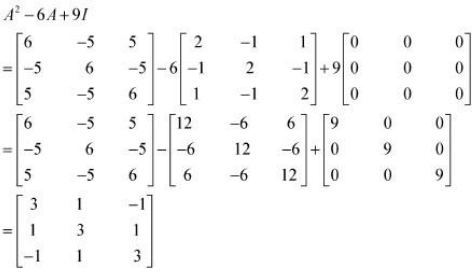From equation (1), we have: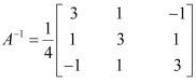Question 17: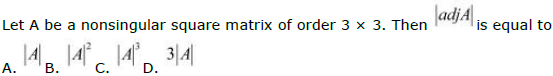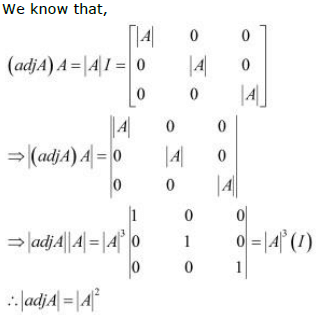Hence, the correct answer is B.

Question 18: If A is an invertible matrix of order 2, then det (A−1) is equal to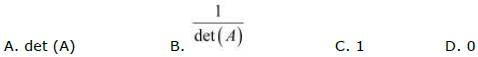B. C. D.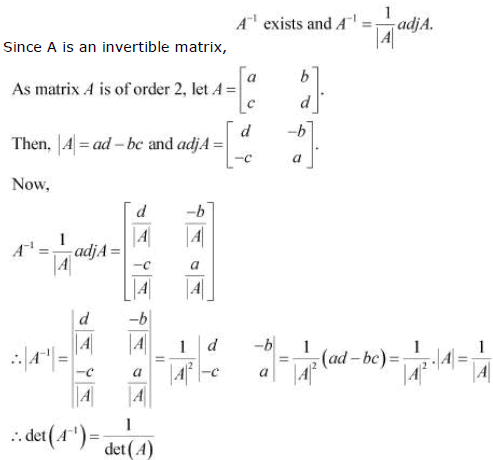Hence, the correct answer is B.

The document NCERT Solutions, Determinants, Part -5, Class 12, Maths, Notes - JEE is a part of JEE category.
All you need of JEE at this link: JEEUse Code STAYHOME200 and get INR 200 additional OFF

Track your progress, build streaks, highlight & save important lessons and more!

,

,

,

,

,

,

,

,

,

,

,

,

,

,

,

,

,

,

,

,

,

,

,

,

,

,

,

,

,

,

,

,

,

,

,

,

;